## Chapter 7 Statistics R.D. Sharma Solutions for Class 10th Math Exercise 7.6

1. 1. Draw an given by less than method for the following data:
No.of rooms: 1  2  3  4  5  6  7  8  9  10
No. of houses: 4  9  22  28  24  12  8  6  5  2

Solution2. The marks scored by 750 students in an examination are given in the form of a frequency distribution table:Solution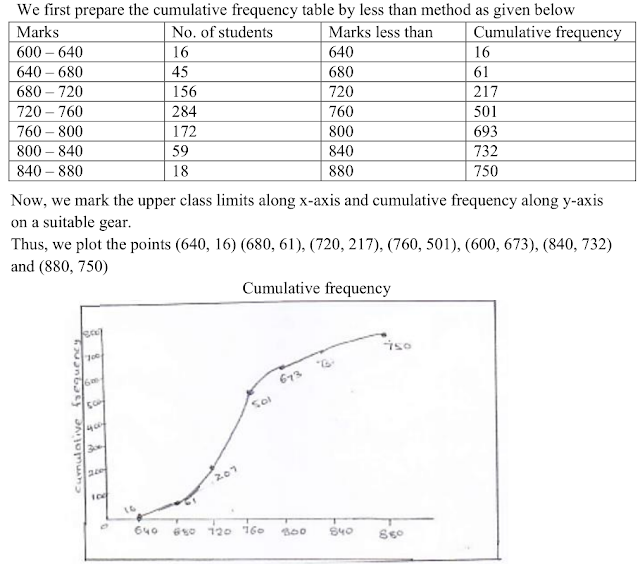3. Draw an ogive to represent the following frequency distribution:
Class-interval: 0-4  5-9  10-14  15-19   20-24
No. of students:  2   6   10   5   3

Solution4. The monthly profits (in Rs.) of 100 shops are distributed as follows:
Profits per shop: 0-50  50-100  100-150  150-200   200-250   250–300
No. of shops:  12  18   27   20  17  6
Draw the frequency polygon for it.

Solution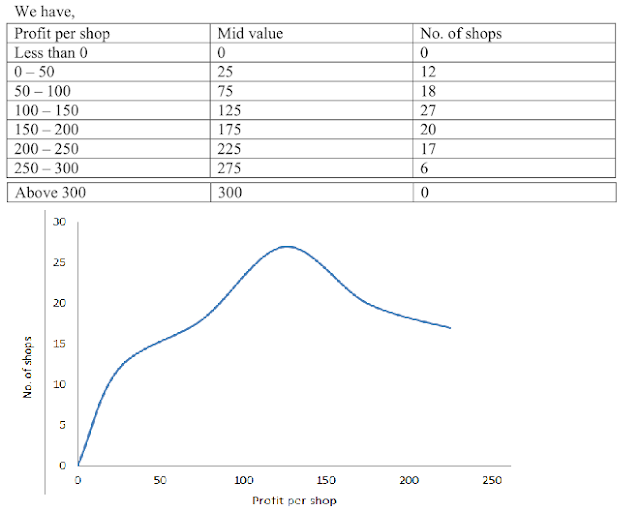5. The  following distribution the daily income of 50 workers  of  a factory :
Daily income in : 100-120  120-140  140-160  160-180  180-200
Number of workers : 12  14   8   6  10
Convert the above distribution to a less than type cumulative frequency distribution and draw its ogive .

Solution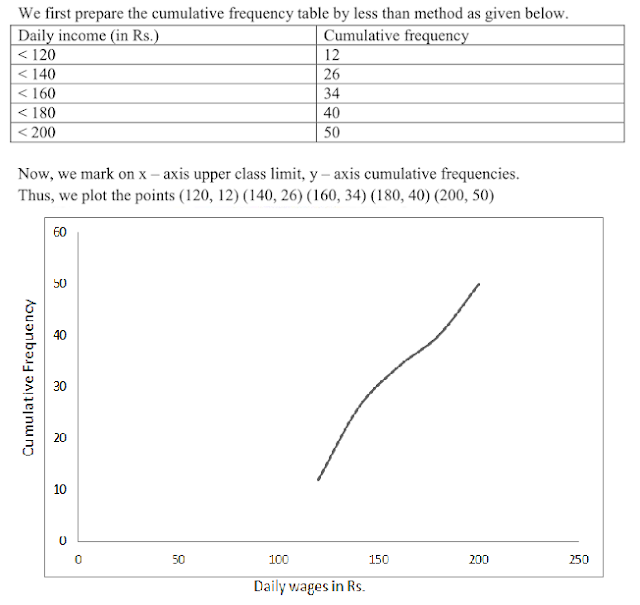6. The following table gives production yield per hectare of wheat of 100 farms of a village:
Production yield in kg per hectare: 50-55  55-60  60-65  65-70  70-75  75-80
Number of farms: 2   8  12   24   38   16
Draw ‘less than’ ogive and ‘more than’ ogive.

Solution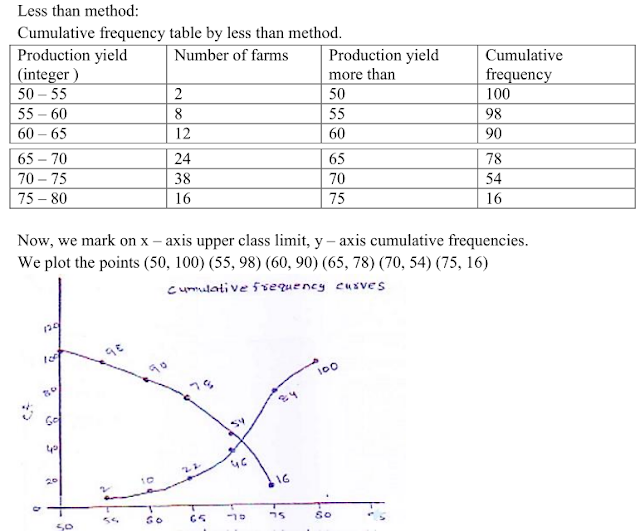7. During the medical check-up of 35 students of a class, their weights were recorded as follows:Draw a less than type ogive for the given data. Hence, obtain the median weight from the
graph and verify the result by using the formula .

Solution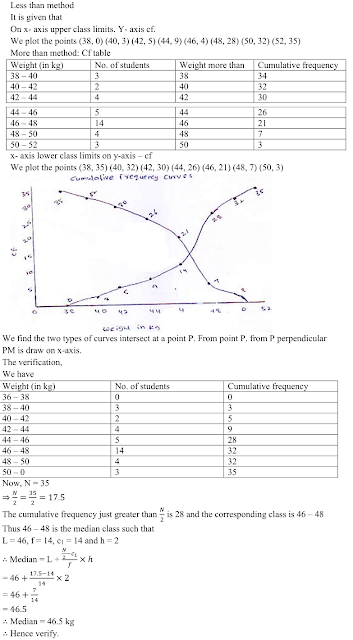8. The annual rainfall record of a city for 66 days is given in the following table :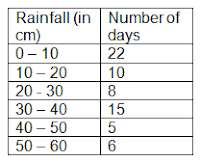Calculate the median rainfall using ogives of more than type and less than type .

Solution

Prepare a table for less than type .
Now, plot the less than ogive using suitable points .Here, N = 66
N/2 = 33
In order to find the median rainfall , we first locate the point corresponding to 33rd day on the y-axis. Let the point be P. From this point draw a line parallel to the x-axis cutting to curve at Q. From this point Q, draw a line parallel to the y - axis and meeting the x-axis at the point R. The x - coordinate of R is 21.25 .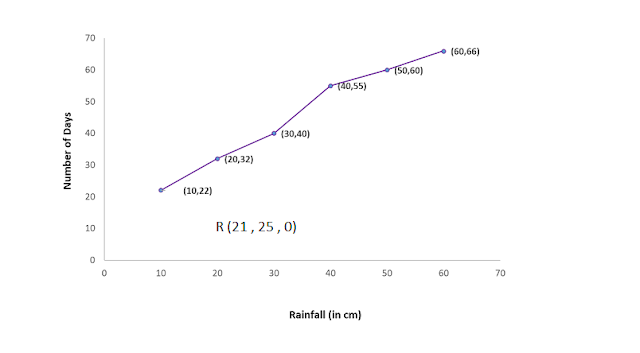Thus, median rainfall is is 21.25 cm .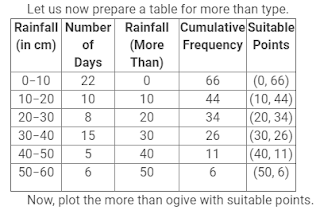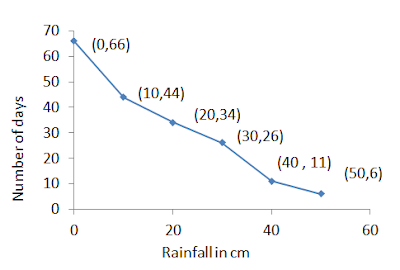Here , N = 66
N/2 =  33
In order to find the median rainfall, we first locate the point corresponding to 33rd day on the y-axis.Let the point be P.From this point Q, draw a line parallel to the y-axis and meeting the x-axis at the point R. The x-coordinates of R is 21.25.

9. The following table gives the height of tress .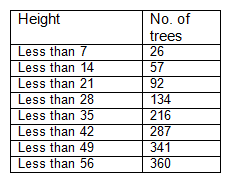Draw ' less than 'ogive and more than 'ogive'

Solution

Consider the following table .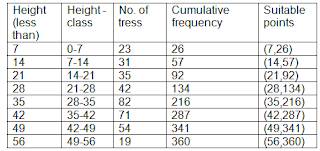Now draw less than ogive using suitable points .Now, prepare the cumulative frequency table for men than series .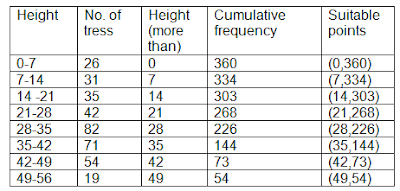Now draw more than ogive using suitable points .10. The annual profits earned by 30 shops of a shopping complex in a locality give rise to the following distribution .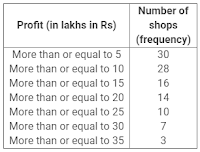Draw both ogives for the above data and hence obtain the median.

Solution

Firstly , we prepare the cumulative frequency table for less than type .Again, prepare the cumulative frequency table for more than type .Now, ''more than ogive'' and ''less than ogive'' can be drawn as follows :The x - coordinate of the point of intersection of the '' more than ogive'' and less- than ogive'' gives the median of the given distribution..
So, the corresponding median is 17.5 lakh .